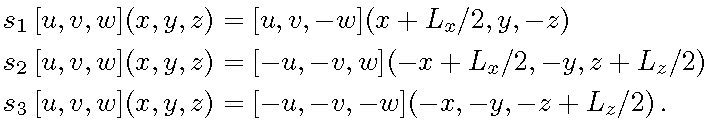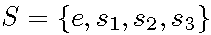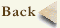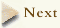## Symmetries of plane Couette flow

 The Navier-Stokes equations for the plane Couette flow are equivariant under "shift-reflect" and "shift-rotate" transformations:By “equivariant” we mean that if u(x,t) is a solution, so are s1u(x,t), s2u(x,t), and s1s2u(x,t); the four solutions are physically equivalent. ( to see examples of such symmetry-related solutions). We refer to the space of velocity fields left invariant under the symmetry groupas the S-invariant subspace. Most of the exact invariant solutions (equilibria, periodic orbits) shown in what follows belong to this subspace; stable/unstable manifolds, travelling waves do not.